# Machine Learning Questions

### By Laura Martinez Molera

These columns will look at a topic, explain some of the background, and answer a few questions that I’ve heard from the MATLAB and Simulink community.

This column is all about model validation, as well as some related topics like overfitting and hyperparameter tuning. I’ll summarize the topic and why it’s important, and then take a look at four questions:

#### 4. How can I improve my model without overfitting it?

Model validation is a foundational technique for machine learning. When used correctly, it will help you evaluate how well your machine learning model is going to react to new data. This is helpful in two ways:

• It helps you figure out which algorithm and parameters you want to use.
• It prevents overfitting during training.

When we approach a problem with a dataset in hand, it is very important that we find the right machine learning algorithm to create our model. Every model has its own strengths and weaknesses. For example, some algorithms have a higher tolerance for small datasets, while others excel with large amounts of high-dimensional data. For this reason, two different models using the same dataset can predict different results and have different degrees of accuracy.

Finding the best model for your data is an interactive process that involves testing out different algorithms to minimize the model error. The parameters that control a machine learning algorithm’s behavior are called hyperparameters. Depending on the values you select for your hyperparameters, you might get a completely different model. So, by tuning the values of the hyperparameters, you can find different, and hopefully better, models.

Without model validation, it is easy to tune your model to the point where it starts overfitting without you realizing it. Your training algorithm is supposed to tune parameters to minimize a loss function, but sometimes it goes too far. When that happens, the model becomes overfit—that is, it’s overly complex and can’t perform well with new data. I’ll tackle this in more depth in the third question.

To test how well your model is going to work with new data, you can use model validation by partitioning a dataset and using a subset to train the algorithm and the remaining data to test it.

Because model validation does not use all of the data to build a model, it is a commonly used method to prevent overfitting during training.

Now, the first question.

If you’d like more details on functions and syntax related to model validation and hyperparameter optimization with MATLAB, see
Model Building and Assessment
.

QUESTION ONE

# My model was working well with the training data, but when I give it new data the results aren’t as good. How do I fix this?

This sounds like you are overfitting your model, which means that your model is completely aligned to the training set but doesn’t know how to respond to new input or data. The model responds “too well” to the dataset you used to train the model.

At the beginning, an overfitted model might seem very promising since the error to the training set is very low. However, the error to the testing set is higher and the model becomes less accurate.

The most common reason for overfitting a model is insufficient training data, so the best solution to this problem is to gather more data and train the model better. But you not only need more data, you also need to make sure this data is representative enough of the model’s complexity and diversity so the model will know how to respond to it.# I know data needs to be split into groups, but I thought the purpose of the testing and training datasets was the same. What’s the difference?

Testing and training datasets are, in fact, different. When I introduced model validation earlier, I talked about how model validation partitions data into these two subsets, so let me dive into that a bit more.

Model validation uses randomly divided data in different subsets to reduce the risk of overfitting a model by tuning the model to respond correctly to new input. The two typical subsets of data are:

• Training set – This data is used to train and fit the model and determine parameters. It is usually 60–70% of the data and needs to reflect the complexity and diversity of the model.
• Testing set – This data is used to evaluate the performance of the model. This is usually about 30–40% of the data, and it also needs to reflect the complexity and diversity of the model.

Since we need to reflect the complexity and diversity of the model in both datasets, they need to be divided randomly. This approach will also decrease the risk of overfitting the model and give us a more accurate but simpler model to produce results for the study.

If we train the model with a non-randomly selected dataset, the model would be trained well for that specific subset of the data. The problem is this non-random data won’t represent the rest of the data or new data that we want to apply the model to. For example, say we are analyzing the energy consumption of a town. If the dataset we use for training and testing is not random and only has data from the weekend energy consumption, which is generally lower than weekdays, when we apply the model to new data such as a new month, it won’t be accurate since it will only represent the weekends.

Let me illustrate this with an example of two models adjusting to a training dataset. I’m going to use a basic example found in the
Machine Learning Onramp
. Here, I have a simple model and a complex model:

### Simple Model

84% Accuracy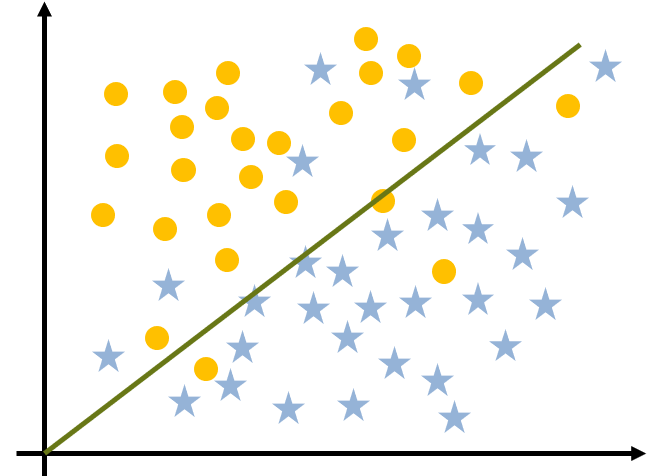### Complex Model

100% Accuracy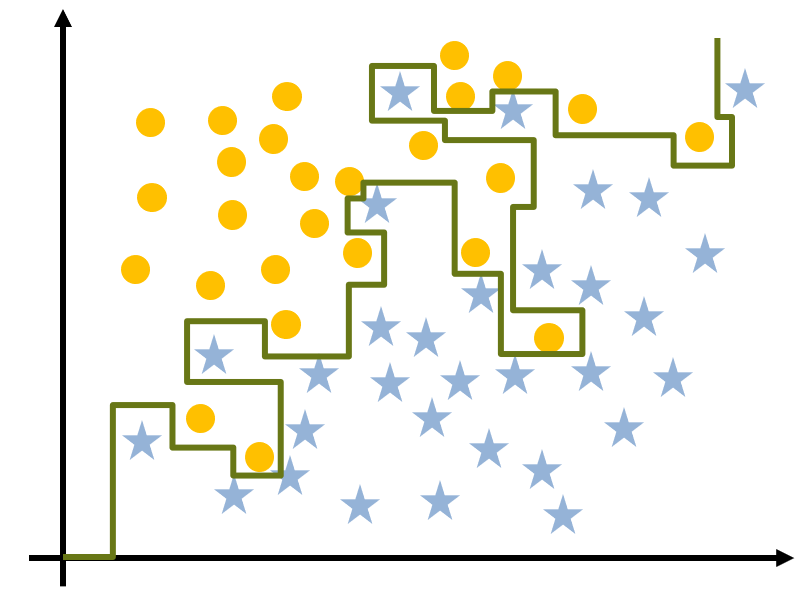You can see that the complex model adapts better to the training data with a performance of a 100% vs. 84% for the simple model. It would be tempting to declare the complex model the winner. However, let’s see the results if I apply the testing dataset (new data that was not used during training) to these models:

### Simple Model

70% Accuracy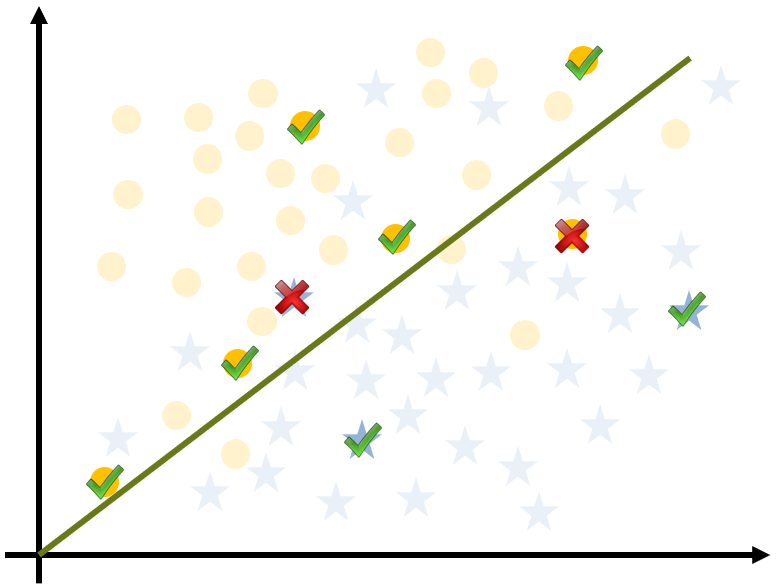### Complex Model

60% Accuracy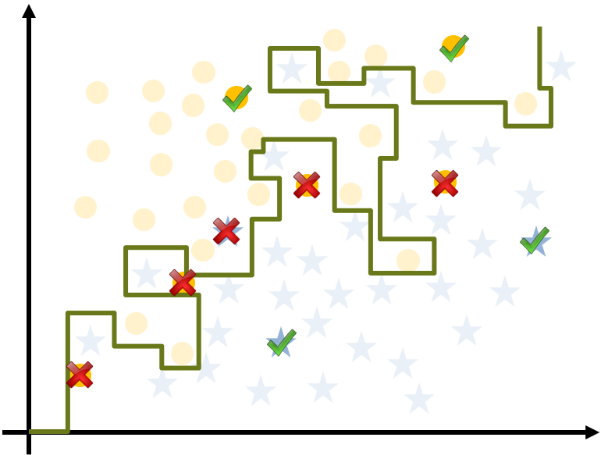When I compare the performance of both models, my simple model’s accuracy has dropped from 84% to 70%; however, that change is much less significant than the 40-point drop seen by the complex model (100% to 60%). In conclusion, the simple model is better and more accurate for this analysis, and it also demonstrates how important it is to have a testing dataset to evaluate the model.

Finally, another recommendation. To reduce variability, do multiple rounds of model validation with different partitions of the dataset to adapt the model better to your analysis. This technique is called k-fold cross-validation
Learn about other techniques for cross-validation
.

The example I used is found in the Machine Learning Onramp. Here’s a clip from that training (2:31):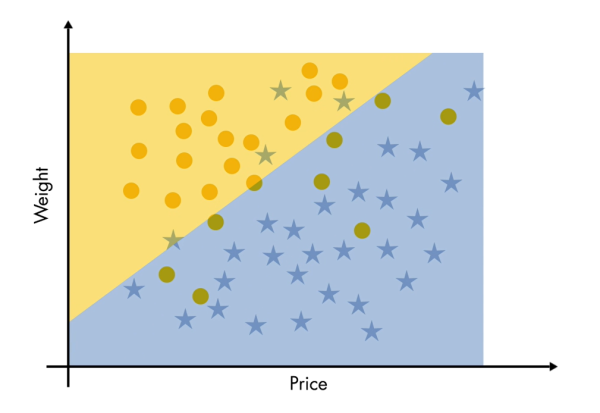# I thought I just needed a training and testing dataset; is having a validation dataset necessary as well? Do I really need to split my data again?

Poor, misunderstood validation set. This is a common question. No one (usually!) questions the need for training and testing sets, but it’s not as clear why we have to partition a validation set as well. The short answer is that validation sets are used when tuning hyperparameters to see whether the tuning is working—in other words, iterating on your complete model. However, sometimes the term validation set is mistakenly used to mean a testing dataset. Here’s a more complete answer for why validation datasets are useful:

• Validation set – This dataset is used to evaluate the performance of the model while tuning the hyperparameters of the model. This data is used for more frequent evaluation and is used to update hyperparameters, so the validation set affects the model indirectly. It is not strictly necessary to tune the hyperparameters of a model, but it’s normally recommended.
• Testing set – This dataset is used to provide an unbiased evaluation of the final model fit in the training set. This set is only used once the model is completely trained and doesn’t affect the model; it’s just to calculate performance.

As a summary, a training dataset trains the different algorithms we have available, and a validation dataset compares performance of the different algorithms (with different hyperparameters) and decides which one to take. A testing dataset gives the accuracy, sensitivity, and performance of the specific model.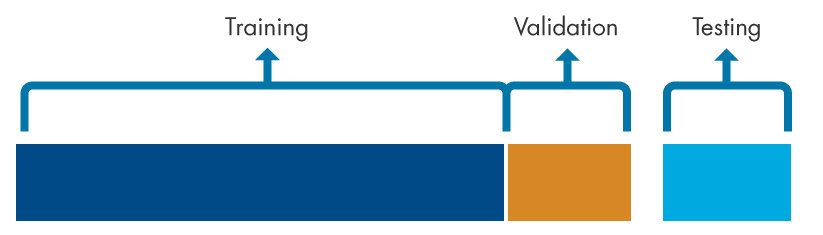## I want to improve my model but I’m afraid of overfitting. What can I do?

This is a great question. In the introduction to this column, I briefly mentioned that hyperparameters control a machine learning algorithm’s behavior. I’ll go into this in a bit more depth now.

You can think of hyperparameters like the components of a bicycle: things we can change that affect the performance of the system. Imagine you buy a used bicycle. The frame is the right size but the bike would probably be more efficient once you’ve adjusted the seat height, tightened or loosened the brakes, oiled the chain, or installed the right tires for your terrain. External factors will also impact your trip, but getting from A to B will be easier with an optimized bike. Similarly, tuning the hyperparameters will help you improve the model.

Now, here’s a machine learning example. In an artificial neural network (ANN), the hyperparameters are variables that determine the structure of the network, such as the number of hidden layers of artificial neurons and the number of artificial neurons in each layer, or variables that define how a model is trained, such as the learning rate, which is the speed of the learning process.

Hyperparameters are defined before the learning process starts. In contrast, the parameters of an ANN are the coefficients or weights of each artificial neuron connection, which are adjusted during the training process.

Typical
neural network
architecture

A hyperparameter is a parameter of a model that is determined before starting the training or learning process and is external to the model; in other words, if you want to change one, you need to manually do it. The bicycle seat won’t adjust itself and you’ll want to do it before setting off; in a machine learning model it would be adjusted with the validation dataset. In contrast, other parameters are determined during the training process with your training dataset.

The time necessary to train and test a model depends on its hyperparameters, and models that have few hyperparameters are easier to validate or adapt, so you could reduce the size of the validation dataset.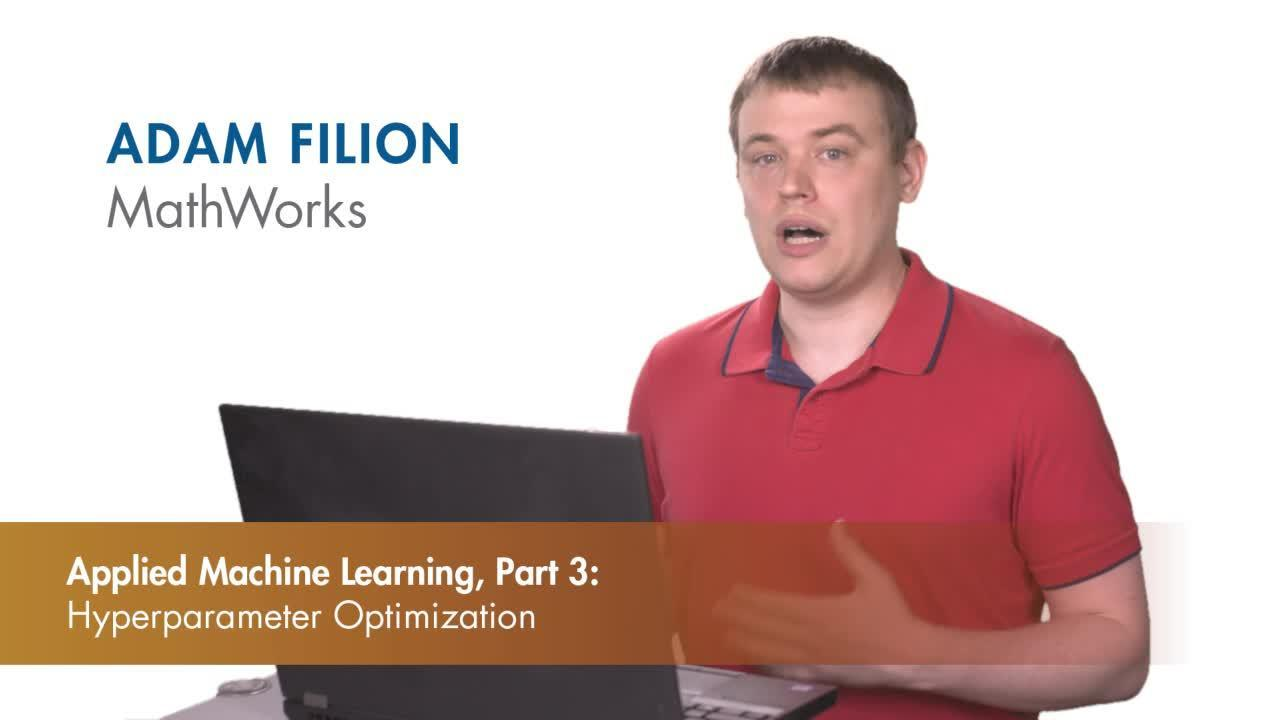Example of Non-convex Surface

Most machine learning problems are non-convex. This means that depending on the values we select for the hyperparameters, we can get a completely different model, and by changing the values of the hyperparameters, we can find different and better models. That’s why the validation dataset is important if you want to iterate with different hyperparameters to find the best model for your analysis.

If you'd like to learn more about hyperparameters, Adam Filion's video (above) on hyperparameter optimization is a great overview in under 5 minutes.